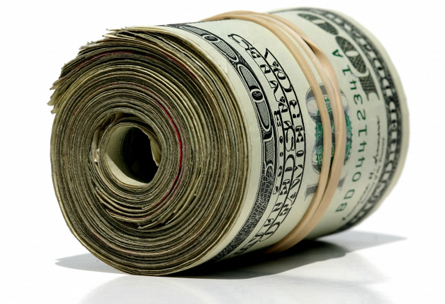## Are you a numeracy expert?In 2006 a numeracy survey was conducted in NZ (and around the world) to determine the distribution of numeracy skills in the adult population.

We didn't do so well...

Anyway, below is the toughest question in the survey.  If you got this (and a few others) you were likely to be placed in the 'expert' level (level five to the L&N folks).

Good luck!

###Problem

You deposit \$1000 into a term deposit that gives a rate of 7% interest per year, and you leave it for ten years.  Assuming nothing else changes, will your money double?

Well, guess what - only about 5% of the population actually managed to get this one.

### Solution

Here is what you need.

Where 'x' is the total and 'p' is the principle, 'i' is the interest rate and 'n' is the years.

x = p (i + 1)^n

Here is a little project for you.  Write this as an excel formula into excel and make it work.  Plug in the numbers above and see if it doubles (right now someones is saying, 'just give me the ----- answer').

### UPDATE:

Okay, here is how you solve it.

Remember Bodmas/bedmas?  Well lets just plug the numbers into the formula and use a calculator.

p = \$1000
i = 7% = .07
n = 10

1.  So, brackets first = (i + 1) = 1.07
2.  Then exponents = 1.07^n = 1.07^10 = 1.97
3.  Then p x 1.07 = 1000 x 1.97 = \$1970

Now most folk get stuck on step 2.  Remember that with exponents you are multiplying the number by itself.  You do this ten times (once for each year).

1.07 x 1.07 x 1.07 x 1.07 x 1.07 x 1.07 x 1.07 x 1.07 x 1.07 x 1.07.  DO NOT do this on paper or in your head.  Grab a scientific calculator and use the  x to the power of y button.  All your phones have this.Just enter 1.07, then push the button, then enter 10, and hey presto!  You have your answer.

Does \$1000 double in ten years at 7% interest?

Now, this is the type of skills that we each need to cut it in the information age.  It's not just about being able to solve this one task, but rather possessing the skills and the courage to actually have a go at it.

If you are happy to just let others do it for you, or to use an existing formula calculator - haven't you given up some of your independence as an adult?

You don't have to know all this perfectly, but do you have the skills to work it out if you want?

Final question:  At what percentage interest point does your money double in ten years?

1.Thanks for sharing, nice post! Post really provice useful information!

An Thái Sơn với website anthaison.vn chuyên sản phẩm máy đưa võng hay máy đưa võng tự động tốt cho bé là địa chỉ bán máy đưa võng giá rẻ tại TP.HCM và giúp bạn tìm máy đưa võng loại nào tốt hiện nay.

1.2.